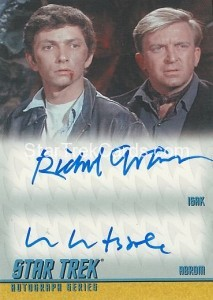Trading Card Details
Trading Card Location
Star Trek: The Original Series → Star Trek: The Remastered Original Series → Double Autographs
Front Middle / Other BackTitle Number Number Made Manufacturer Material Size
Richard Evans & William Wintersole DA11 300-500 Rittenhouse Archives Cardboard / Paper 2½” x 3½” (Standard)
Year Original Art Autograph Costume / Relic Sticker Die Cut
2009 No Yes No No No
Notes
None

Non-Graded Price History Averages
1 Month 3 Months 6 Months 1 Year 3 Years Lifetime
[insert_php] \$counter = 0; \$price=0; [/insert_php][insert_php] if(strtotime('September 20, 2015')>strtotime('-1 months')){ \$counter++; \$price = \$price + 27.04; } [/insert_php][insert_php] if(strtotime('January 4, 2016')>strtotime('-1 months')){ \$counter++; \$price = \$price + 23.00; } [/insert_php][insert_php] if(strtotime('January 30, 2016')>strtotime('-1 months')){ \$counter++; \$price = \$price + 12.38; } [/insert_php][insert_php]; if (\$counter > 0) { //checks to make sure there won't be a divide by zero error \$totalaverage = (\$price / \$counter); //divide monetary sum by the number of entries if (\$_GET['item_number'] != \$_GET['reference']) { \$totalaverage = (\$totalaverage / \$_GET['num']); } } echo '\$'; //echo the dollar sign round (\$totalaverage, 2); //round the number to a maximum of two decimal places echo number_format((float)\$totalaverage, 2, '.', ''); //force two decimal places for monetary consistency [/insert_php] [insert_php] \$counter = 0; \$price=0; [/insert_php][insert_php] if(strtotime('September 20, 2015')>strtotime('-3 months')){ \$counter++; \$price = \$price + 27.04; } [/insert_php][insert_php] if(strtotime('January 4, 2016')>strtotime('-3 months')){ \$counter++; \$price = \$price + 23.00; } [/insert_php][insert_php] if(strtotime('January 30, 2016')>strtotime('-3 months')){ \$counter++; \$price = \$price + 12.38; } [/insert_php][insert_php]; if (\$counter > 0) { //checks to make sure there won't be a divide by zero error \$totalaverage = (\$price / \$counter); //divide monetary sum by the number of entries if (\$_GET['item_number'] != \$_GET['reference']) { \$totalaverage = (\$totalaverage / \$_GET['num']); } } echo '\$'; //echo the dollar sign round (\$totalaverage, 2); //round the number to a maximum of two decimal places echo number_format((float)\$totalaverage, 2, '.', ''); //force two decimal places for monetary consistency [/insert_php] [insert_php] \$counter = 0; \$price=0; [/insert_php][insert_php] if(strtotime('September 20, 2015')>strtotime('-6 months')){ \$counter++; \$price = \$price + 27.04; } [/insert_php][insert_php] if(strtotime('January 4, 2016')>strtotime('-6 months')){ \$counter++; \$price = \$price + 23.00; } [/insert_php][insert_php] if(strtotime('January 30, 2016')>strtotime('-6 months')){ \$counter++; \$price = \$price + 12.38; } [/insert_php][insert_php]; if (\$counter > 0) { //checks to make sure there won't be a divide by zero error \$totalaverage = (\$price / \$counter); //divide monetary sum by the number of entries if (\$_GET['item_number'] != \$_GET['reference']) { \$totalaverage = (\$totalaverage / \$_GET['num']); } } echo '\$'; //echo the dollar sign round (\$totalaverage, 2); //round the number to a maximum of two decimal places echo number_format((float)\$totalaverage, 2, '.', ''); //force two decimal places for monetary consistency [/insert_php] [insert_php] \$counter = 0; \$price=0; [/insert_php][insert_php] if(strtotime('September 20, 2015')>strtotime('-1 year')){ \$counter++; \$price = \$price + 27.04; } [/insert_php][insert_php] if(strtotime('January 4, 2016')>strtotime('-1 year')){ \$counter++; \$price = \$price + 23.00; } [/insert_php][insert_php] if(strtotime('January 30, 2016')>strtotime('-1 year')){ \$counter++; \$price = \$price + 12.38; } [/insert_php][insert_php]; if (\$counter > 0) { //checks to make sure there won't be a divide by zero error \$totalaverage = (\$price / \$counter); //divide monetary sum by the number of entries if (\$_GET['item_number'] != \$_GET['reference']) { \$totalaverage = (\$totalaverage / \$_GET['num']); } } echo '\$'; //echo the dollar sign round (\$totalaverage, 2); //round the number to a maximum of two decimal places echo number_format((float)\$totalaverage, 2, '.', ''); //force two decimal places for monetary consistency [/insert_php] [insert_php] \$counter = 0; \$price=0; [/insert_php][insert_php] if(strtotime('September 20, 2015')>strtotime('-3 years')){ \$counter++; \$price = \$price + 27.04; } [/insert_php][insert_php] if(strtotime('January 4, 2016')>strtotime('-3 years')){ \$counter++; \$price = \$price + 23.00; } [/insert_php][insert_php] if(strtotime('January 30, 2016')>strtotime('-3 years')){ \$counter++; \$price = \$price + 12.38; } [/insert_php][insert_php]; if (\$counter > 0) { //checks to make sure there won't be a divide by zero error \$totalaverage = (\$price / \$counter); //divide monetary sum by the number of entries if (\$_GET['item_number'] != \$_GET['reference']) { \$totalaverage = (\$totalaverage / \$_GET['num']); } } echo '\$'; //echo the dollar sign round (\$totalaverage, 2); //round the number to a maximum of two decimal places echo number_format((float)\$totalaverage, 2, '.', ''); //force two decimal places for monetary consistency [/insert_php] [insert_php] \$counter = 0; \$price=0; [/insert_php][insert_php] \$counter++; \$price = \$price + 27.04; [/insert_php][insert_php] \$counter++; \$price = \$price + 23.00; [/insert_php][insert_php] \$counter++; \$price = \$price + 12.38; [/insert_php][insert_php]; if (\$counter > 0) { //checks to make sure there won't be a divide by zero error \$totalaverage = (\$price / \$counter); //divide monetary sum by the number of entries if (\$_GET['item_number'] != \$_GET['reference']) { \$totalaverage = (\$totalaverage / \$_GET['num']); } } echo '\$'; //echo the dollar sign round (\$totalaverage, 2); //round the number to a maximum of two decimal places echo number_format((float)\$totalaverage, 2, '.', ''); //force two decimal places for monetary consistency [/insert_php]

Price History Detail
Date Sold Price Graded Condition Location User
January 30, 2016 \$12.38 No Near Mint/Mint (7-10) Ebay admin
January 4, 2016 \$23.00 No Near Mint/Mint (7-10) Ebay admin
September 20, 2015 \$27.04 No Near Mint/Mint (7-10) Ebay admin